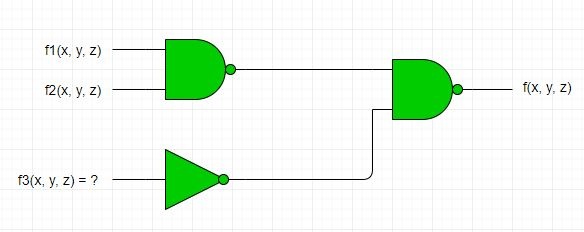# GATE | GATE-CS-2002 | Question 50

Consider the following logic circuit whose inputs and function and output is f.Given that

```f1(dx, y, z) = ∑(0, 1, 3, 5),
f2(dx, y, z) = ∑(6, 7) and
f(dx, y, z) = ∑(1, 4, 5),```

f3 is :
(A) ∑(1, 4, 5)
(B) ∑(6, 7)
(C) ∑(0, 1, 3, 5)
(D) None of these

Explanation: Function f will be:

`f = ((f1f2)′(f3)')′ = (f1f2) + f3`

Since AND of two expressions will contain the common terms and f1 and f2 don’t have any common term, so f1f2 is 0 and hence f = f3 = Σ(1,4,5).

Option (A) is correct.

Quiz of this Question